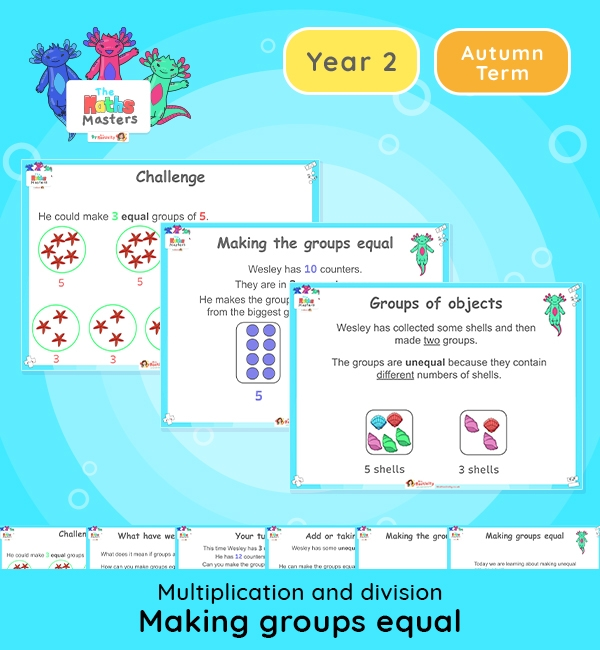Year 2 | Making Groups Equal Lesson PresentationWhite Rose Year multiplication and division resources

Aligned with the White Rose Maths scheme of work, this Year 2 | Making Groups Equal Lesson Presentation is fully editable, and is designed for the Year 2 maths curriculum covering the following maths objectives for the autumn term:

Topic: Multiplication and division

This lesson focuses on making unequal groups equal.

White Rose Maths Small Steps: Make equal groups

NC Links: Recall and use multiplication and division facts for the 2,5, and 10 times tables. *solve problems involving multiplication and division using materials, array, repeated addition, mental methods and multiplication and division facts including problems in context.

TAF Statements:– Recall and use multiplication and division facts for 2, 5, and 10 and use them to solve simple problems. Working At

Greater Depth – Use reasoning about number and relationships to solve more complex problems and explain their thinking.

Year 1 conceptual prerequisites: Count in multiples of 2, 5 and 10.

Explore our other year 2 multiplication and division resources.